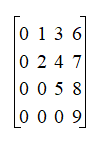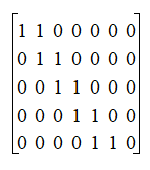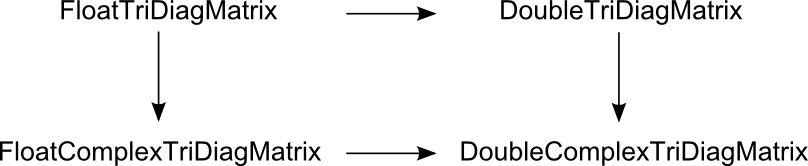# NMath User's Guide

18.1 Creating Matrices (.NET, C#, CSharp, VB, Visual Basic, F#)

This section describes how to create instances of the structured sparse matrix classes.

Creating Default Matrices

You can construct default structured sparse matrices by supplying the necessary parameters to describe the matrix shape, as shown in Table 12. All stored values are initialized to zero.

 Matrix Type Shape Parameters Lower Triangular Order Upper Triangular Order Symmetric Order Hermitian Order Banded Rows, Columns, Lower Bandwidth, Upper Bandwidth TriDiagonal Rows, Columns Symmetric Banded Order, Half Bandwidth Hermitian Banded Order, Half Bandwidth

Square matrix types are characterized by their order--that is, the number of rows and columns. For example, a matrix of order 3 is a 3 x 3 matrix. Thus, this code creates a default 5 x 5 Hermitian matrix of double-precision complex numbers:

Code Example – C# matrix

```var A = new DoubleHermitianMatrix( 5 );
```

Code Example – VB matrix

```Dim A As New DoubleHermitianMatrix(5)
```

Constructors for rectangular matrix types accept separate row and column shape parameters. For example:

Code Example – C# matrix

```var A = new DoubleTriDiagMatrix ( 3, 5 );
```

Code Example – VB matrix

```Dim A As New DoubleTriDiagMatrix(3, 5)
```

Constructors for banded matrix types also accept bandwidth parameters that describe the width of the banded region. Thus, the following code creates a 4 x 5 FloatComplexBandMatrix with a lower bandwidth of 1 and an upper bandwidth of 2:

Code Example – C# matrix

```var A = new FloatComplexMatrix( 4, 5, 1, 2 );
```

Code Example – VB matrix

```Dim A As New FloatComplexMatrix(4, 5, 1.0F, 2.0F)
```

This creates an 8 x 8 FloatSymBandMatrix with a half bandwidth of 2:

Code Example – C# matrix

```var A = new FloatSymBandMatrix( 8, 2 );
```

Code Example – VB matrix

```Dim A As New FloatSymBandMatrix(8, 2)
```

Once you've constructed a default matrix, you can set individual values using the provided indexers (Section 18.2). In some case, methods are also provided that return vector views of the underlying data, which can also be used to set matrix values (Section 18.5).

Creating Sparse Matrices from General Matrices

You can construct all NMath structured sparse matrix types from general matrix types. Such constructors extract the appropriate values from the general matrix. Data is copied.

For example, this code constructs a FloatUpperTriMatrix instance by extracting the upper triangular region of a square general matrix:

Code Example – C# matrix

```var genMat = new FloatMatrix( 5, 5, 0, 1 );
```
```var A = new FloatUpperTriMatrix( genMat );
```

Code Example – VB matrix

```Dim GenMat As New FloatMatrix(5, 5, 0.0F, 1.0F)
```
```Dim A As New FloatUpperTriMatrix(GenMat)
```

Constructors for square matrix types, such as upper triangular matrices, throw a MatrixNotSquareException if the given general matrix is not square. Alternatively, you can pass in a non-square general matrix and specify the order of the square submatrix to extract. Thus, this code creates a 3 x 3 DoubleSymmetricMatrix by extracting the upper triangular region of the 3 x 3 leading submatrix from the given 4 x 6 general matrix:

Code Example – C# matrix

```var genMat = new DoubleMatrix( 4, 6, 0, 0.25 );
```
```var A = new DoubleSymmetricMatrix( A, 3 );
```

Code Example – VB matrix

```Dim GenMat As New DoubleMatrix(4, 6, 0.0, 0.25)
```
```Dim A As New DoubleSymmetricMatrix(A, 3)
```

An IndexOutOfRangeException is raised if the given order specifies a submatrix that is out of bounds.

Banded matrix types can also be constructed from general matrices by specifying the desired bandwidth. For instance, the following code extracts the values required to construct a Hermitian banded matrix with a half bandwidth of 3 from the given general matrix:

Code Example – C# matrix

```var incr = new DoubleComplex( 1, 0.25 );
```
```var genMat = new DoubleComplexMatrix( 12, 12, 0, incr );
```
```var A = new DoubleHermitianBandMatrix( A, 3 );
```

Code Example – VB matrix

```Dim Incr As New DoubleComplex(1.0, 0.25)
```
```Dim GenMat As New DoubleComplexMatrix(12, 12, 0.0, Incr)
```
```Dim A As New DoubleHermitianBandMatrix(A, 3)
```

Creating Sparse Matrices from Other Sparse Matrices

Some structured sparse matrix types can be constructed from other structured sparse matrices. For example, a tridiagonal matrix is really a special case of a banded matrix with lower and upper bandwidth equal to 1. Therefore, banded matrices can be constructed from tridiagonal matrices, and vice versa. For example:

Code Example – C# matrix

```int rows = 8, cols = 8, ub = 0, lb = 2;
```
```var data = new FloatVector( (ub+lb+1)*cols, 1, 1 );
```
```var A = new FloatBandMatrix( data, rows, cols, lb, ub );
```
```var B = new FloatTriDiagMatrix( A );
```

Code Example – VB matrix

```Dim Rows As Integer = 8
```
```Dim Cols As Integer = 8
```
```Dim UB As Integer = 0
```
```Dim LB As Integer = 2
```
```Dim Data As New FloatVector((UB + LB + 1) * Cols, 1.0F, 1.0F)
```
```Dim A As New FloatBandMatrix(Data, Rows, Cols, LB, UB)
```
```Dim B As New FloatTriDiagMatrix(A)
```

Similarly, you can construct banded matrices from symmetric or Hermitian banded matrices, or triangular matrices from symmetric or Hermitian matrices, and vice versa.

Creating Sparse Matrices from a Data Vector

You can construct all NMath structured sparse matrix types from an appropriate data vector and shape parameters. The vector storage scheme used by each structured sparse matrix type is described in Chapter 17. For example, you could create this 4 x 4 symmetric matrix:like this:

Code Example – C# matrix

```var data = new DoubleVector( 10, 0, 1 );
```
```var A = new DoubleSymmetricMatrix( data, 4 );
```

Code Example – VB matrix

```Dim Data As New DoubleVector(10, 0.0, 1.0)
```
```Dim A As New DoubleSymmetricMatrix(Data, 4)
```

Similarly, you could create this 5 x 7 banded matrix with an upper bandwidth of 1 and a lower bandwidth of 0:using this code:

Code Example – C# matrix

```var data = new FloatVector( 14, 1 );
```
```var A = new FloatBandMatrix( data, 5, 7, 0, 1 );
```

Code Example – VB matrix

```Dim Data As New FloatVector(14, 1.0F)
```
```Dim A As New FloatBandMatrix(Data, 5, 7, 0, 1)
```

Implicit Conversion

NMath provides implicit conversion operators for the structured sparse matrix classes. Single-precision types are implicitly promoted to double-precision types, and real types are implicitly promoted to complex types, as shown in Figure 4. An arrow indicates implicit promotion.

Figure 4 – Implicit conversion for matrix data typesFor example, Figure 5 shows the pattern for implicit conversion among the tridiagonal types.

Figure 5 – Implicit conversion for tridiagonal matricesCopying Matrices

The NMath structured sparse matrix classes provide three copy methods:

Clone() returns a deep copy of a matrix. Data is copied; each matrix references different data.

ShallowCopy() returns a shallow copy of a matrix. Data is not copied; both matrices reference the same data.

DeepenThisCopy() copies the data viewed by a matrix to new data block. This guarantees that there is only one reference to the underlying data, and that this data is in contiguous storage.

For instance:

Code Example – C# matrix

```var A = new FloatUpperTriMatrix( 5 );
```
```FloatUpperTriMatrix B = A.ShallowCopy();

```

```B[0,0] = 1;   // A[0,0] == B[0,0]
```
```B.DeepenThisCopy();
```
```B[0,0] = 2;   // A[0,0] != B[0,0]
```

Code Example – VB matrix

```Dim A As New FloatUpperTriMatrix(5)
```
```Dim B As FloatUpperTriMatrix = A.ShallowCopy()

```

```B(0, 0) = 1   ' A[0,0] == B[0,0]
```
```B.DeepenThisCopy()
```
```B(0, 0) = 2   ' A[0,0] != B[0,0]
```

Top

Top